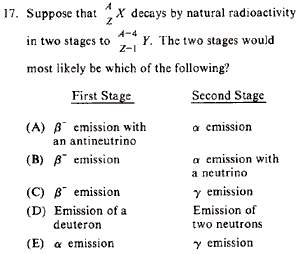GR 8677927796770177 | # Login | Register

GR8677 #17
Problem
 GREPhysics.NET Official Solution Alternate Solutionsby natural decay
Recall the following decay processes, and recall that the subscripts and superscripts have to balance on both sides of the arrow.

Beta () Decay:

where is the symbol of an anti-neutrino. (The decay is accompanied by a neutrino, , instead of an anti-neutrino.)

Alpha Decay:

Gamma Decay (no change in A or Z):

Deuteron Decay (rare):

(A) . This works out.

(B) decay always occurs with an antineutrino.

(C) emission doesn't change the atomic number.

(D) Although the numbers work out here, deuteron decay is rare, thus not natural.

(E) The subscripts and superscripts don't add up.Alternate Solutions
 There are no Alternate Solutions for this problem. Be the first to post one!
jmason86
2009-08-12 21:37:41
I believe ETS made a typo in the problem statement. The subscript on Y should be Z+1 to be consistent with the rest of the problem.
That is because if Z (the # of protons) decreases by 1, it implies a proton turning into a neutron ( decay). To conserve charge you must emit a positron and to conserve lepton number (from the positron) you must also emit an electron neutrino.

In short, p -> n + e

Correct me if I'm wrong :)
 segfault2009-08-22 22:06:46 After the *first* stage in the process the subscript should be Z+1, but after alpha emission the subscript becomes Z+1-2=Z-1. This is a two step process.You are replying to:
I believe ETS made a typo in the problem statement. The subscript on Y should be Z+1 to be consistent with the rest of the problem. That is because if Z (the # of protons) decreases by 1, it implies a proton turning into a neutron ( decay). To conserve charge you must emit a positron and to conserve lepton number (from the positron) you must also emit an electron neutrino. In short, p -> n + e Correct me if I'm wrong :)LaTeX syntax supported through dollar sign wrappers $, ex.,$\alpha^2_0$produces . type this... to get...$\int_0^\infty\partial\Rightarrow\ddot{x},\dot{x}\sqrt{z}\langle my \rangle\left( abacadabra \right)_{me}\vec{E}\frac{a}{b}\$